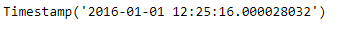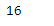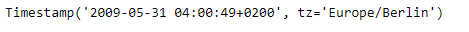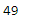GeeksforGeeks App
Open AppBrowser
Continue

# Python | Pandas Timestamp.second

Python is a great language for doing data analysis, primarily because of the fantastic ecosystem of data-centric python packages. Pandas is one of those packages and makes importing and analyzing data much easier.

Pandas` Timestamp.second` attribute return an integer value which represents the value of second in the given Timestamp object.

Syntax : Timestamp.second

Parameters : None

Return : second

Example #1: Use `Timestamp.second` attribute to find second’s value in the given Timestamp object.

 `# importing pandas as pd``import` `pandas as pd`` ` `# Create the Timestamp object``ts ``=` `pd.Timestamp(``2016``, ``1``, ``1``, ``12``, ``25``, ``16``, ``28``, ``32``)`` ` `# Print the Timestamp object``print``(ts)`

Output :Now we will use the `Timestamp.second` attribute to find the second’s value in the given object.

 `# return the value of second``ts.second`

Output :As we can see in the output, the `Timestamp.second` attribute has returned 16 indicating that the second’s value in the given object is set to 16.

Example #2: Use `Timestamp.second` attribute to find second’s value in the given Timestamp object.

 `# importing pandas as pd``import` `pandas as pd`` ` `# Create the Timestamp object``ts ``=` `pd.Timestamp(year ``=` `2009``,  month ``=` `5``, day ``=` `31``, hour ``=` `4``, ``                            ``second ``=` `49``, tz ``=` `'Europe/Berlin'``)`` ` `# Print the Timestamp object``print``(ts)`

Output :Now we will use the `Timestamp.second` attribute to find the second’s value in the given object.

 `# return the value of second``ts.second`

Output :As we can see in the output, the `Timestamp.second` attribute has returned 49 indicating that the second’s value in the given object is set to 49.

My Personal Notes arrow_drop_up